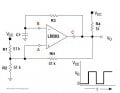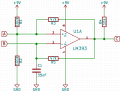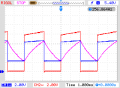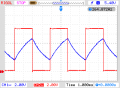# Square wave oscillator using LM393 comparator

#### hrs

Joined Jun 13, 2014
388
Hi,

After breadboarding a square wave oscillator according to the attached circuit diagram, I measured it at slightly over 11 kHz at 2.5 V peak to peak. If I do the frequency calculation with R3 = 1k and C1 = 35nF(measured) 11 kHz is about right.

I don't understand the operation of the circuit. Suppose that at some point in time C1 is discharged and the output just went high, C1 will now charge through R3 until the voltage at B is higher than the voltage at A (4.5 V). Now the output goes low and starts to drain C1. Pretty much immediately the voltage at B will be lower than the voltage at A and the output will go high again, oscillation occurring at infinite Hz. But it doesn't. Why not?

Looking at the functional block diagram on page 9, I think for logical low output pin 1 sinks current to the potential on pin 4. For logical high the output transistor is cut off and a pull-up resistor pulls the output up, to 9 V in my case. So where does the current come from that charges C1? It can't be through the pull-up resistor because it would change the frequency by a lot.

Why am I only seeing 2.5 V peak to peak and not 9 V? I'm not sure if my scope is 1M or 2M ohm input impedance, but with my 10M ohm multimeter I see the same voltage. I'm not seeing the attenuator if there is one.

#### Attachments

• 7.8 KB Views: 212
• 880 KB Views: 44

#### mcasale

Joined Jul 18, 2011
210
The LM393 has an open-collector output. So when the output is HIGH (ie open circuit) the capacitor charges through R3+R4. The voltage at C is almost the same as B.

When the capacitor voltage (B) hits 4.5 volts the comparator output sinks current and discharges the capacitor through R3 only.

So, the charge and discharge time-constants are different. That means the resulting output signal will have a DC offset.

If you want the output to go from ground to 9V (almost), you need to set the time-constants long enough to allow full charge and discharge times. Try swapping R3 and R4 and it will be more symmetrical.

#### hrs

Joined Jun 13, 2014
388
Thanks mcasale, I'll try some different values for R3 and R4. So the charging does occur through R4. Then I need to reconsider how the frequency is calculated. Also it just occurred to me that I need to look at B vs GND on my scope to see what it looks like, tomorrow.

Still I don't understand how C1 gets time to discharge. Suppose A is fixed at 4.5. B rises to 4.50000000000001. Output goes low. In no time B falls to 4.499999999999999999999. Output goes high. Etc. But this is not what happens. Maybe if I scope B to GND I'll get some clues.

#### mcasale

Joined Jul 18, 2011
210
Yes, you are correct, I think. Now that I look more carefully, pin 2 should oscillate around 4.5 volts, and its amplitude is determined by the offset voltage between pins 2 and 3. The capacitor will not totally discharge (or charge), but will operate almost linearly at a fraction of the time constant. In order to get a full 9V output, try switching to a non-open-collector comparator. Then the output will switch between 0 and 9 volts.

Still, the charge and discharge time constants are different, so it will not be symmetrical.

What are you trying to do with this circuit?

#### AnalogKid

Joined Aug 1, 2013
10,592
This is not the standard opamp squarewave oscillator. It is missing a hysteresis resistor from the output to the + input. If you install a 1 M as a starting value, that will be 5% hysteresis, probably enough to get things to behave. The output now will swing the down to GND. It still won't go all the way up to Vcc, but it will be closer. Also, the frequency probably will decrease. As you decrease the hysteresis resistor the frequency will continue to lower.

Having an asymmetrical output impedance makes calculating the theoretical output freq more difficult, and as mentioned the output will not be symmetrical.

ak

#### Jony130

Joined Feb 17, 2009
5,457
It's really hard to believe that this circuit indeed is oscillating. As point out by AnalogKid this circuit is not a standard opamp squarewave oscillator.
Because the "standard circuit" use a hysteresis to generate a square wave. But in your circuit we do not have any hysteresis resistor (RH resistor in the diagram below).And this means that if your circuit really osculating this oscillation are caused by a phase shift in the negative feedback loop. And your circuit on the breadboard fulfils the condition needed to start oscillations. So you are very lucky man.

Also as a side note, your LM393 has an open-collector output so it is always good to choose R4 (pull-up resistor) much smaller in value than the rest of the resistors connected to the output. So, R4 should have the smallest value in the circuit R4 << R3 and RH

Last edited:

#### dannyf

Joined Sep 13, 2015
2,197
But it doesn't. Why not?
Because the 'opamp' here has an open collector output so the charging up is done via a current source formed by vcc and r4.

To put it the other way, had you used a real opamp with a pp output stage, the oscillation would be happening at much higher frequencies for exactly the reason you mentioned.

This oscillator is equivalent to is rc oscillators built with CMOS input stage. The suggested changes would make it similar to rc oscillators built from a st input stage.

#### hrs

Joined Jun 13, 2014
388
Doh! I see now that I forgot a resistor between the non-inverting input and the output in the diagram ... On my breadboard there is a 47 kOhm resistor between pin 1 and 3, I was looking at an incorrect diagram. So the non-inverting input does not simply sit at 4.5 V, it will shift with the output through the hysteresis resistor.

•DickCappels

#### hrs

Joined Jun 13, 2014
388
After some corrective circuitry I tried to re-analyse the oscillator and I was hoping that someone might look at it to see if the if it's any good. I tried to look at the general operation and what influences the output signal voltages. When I have more time I'll try to figure out why it's not oscillating for various values.When V_input < V_inverting_input, the comparator output is shorted to ground:R_parallel = R2*R3/(R2+R3)
V_A = V_bat * R_parallel / (R1 + R_parallel) = 0.1V
V_C = 0V

The voltage at B will drop to V_A. At this point the comparator output transistor goes open circuit.Remove R3 and find Thevenin's equivalent resistance, the current through R3:V_A = V_bat * R2 / (R1 + R2)
V_B = V_bat * R5 / (R4 + R5)
dV_Thev = V_B - V_A
R_Thev = 1/(1/R1 + 1/R2) + 1/(1/R4 + 1/R5)

I3 = dV_Thev / (R_Thev + R3)
V_AB = dV_Thev * R3 / (R_Thev + R3)

The current through R2 = I1 + I3:
V_bat - I3*R2 = I1*R1 + I1*R2
I1 = (V_bat - I3*R2) / (R1 + R2)

V_A = (I1 + I3)*R2
V_C = V_A + V_AB = 7.0V

In conclusion the above suggests a peak to peak voltage at C of 7V. On a scope I see about 3V. If you have any ideas on this I'd love to hear it.

#### RichardO

Joined May 4, 2013
2,270
The peak to peak voltage is not the important value. The positive feedback sets thresholds that the capacitor charges and discharges between. What is important is whether the capacitor can truly get to both of these voltages.

Your equivalent circuits are not quite. They only apply during startup and/or if the circuit does not oscillate. When the circuit is oscillating, the peak voltages on the capacitor are set by the positive feedback. The capacitor-end of R5 never goes to +9 volts or ground. This means that the current through the R5 is less than you estimated.

Also as a side note, your LM393 has an open-collector output so it is always good to choose R4 (pull-up resistor) much smaller in value than the rest of the resistors connected to the output. So, R4 should have the smallest value in the circuit R4 << R3 and RH
My mental calculations say that the circuit is marginal. It may or may not oscillate with real world tolerances of components. As mentioned earlier, the major cause of this is the too-high value of the pullup resistor, R4.

One goal of engineering is to keep things simple. By simply keeping the pullup resistor small relative to the loads it has to drive most of your analysis is unneeded. Also, by keeping the thresholds small relative to the supply voltage, the loading on the pullup resistor becomes less critical.

There are limits to how large and small R4 can be. The LM393 can only sink a limited amount of current and your battery will last longer with larger resistor values. Conversely, if R4 is too large, then the feedback resistors can be too large relative to the input resistance/bias currents of the LM393 and the leakage current of the timing capacitor.

•hrs

#### Jony130

Joined Feb 17, 2009
5,457
Va at time when comparator output transistor goes open circuit is equal to
Va = Vcc * R2/(R1|| (R3+R4) + R2) = 9V * 100kΩ/ (100kΩ||57kΩ + 100kΩ) = 9V* 100kΩ/ (36.3kΩ+ 100kΩ) ≈ 6.6V
In real life this voltage will be smaller due to loading effect by R5. (try lower R4).

Va at time when comparator output is shorted to ground

Va ≈ Vcc* (R2||R3)/( (R2||R3) + R1) = 9V*32kΩ/(132kΩ) ≈ 2.1V (I ignore LM393 saturation voltage)

This sill give us the output frequency equal to Tlow+Thigh:

Tlow ≈ 47kΩ*35nF * ln(6.6/2.1) = 1.88ms . The capacitor is discharging from 6.6V to 2.1V via R5 resistor.

Thigh ≈ (47kΩ+10kΩ)*35nF *ln [ (9 - 2.1) / (9 - 6.6) ] = 2.1ms I ignore R5 resistor influence.
And this time the cap is charging from 2.1V to 6.6V via R4+R5

Fout = 1/(Tlow + Thigh) = 251Hz

And tomorrow I will try to build this circuit I see if theory agrees with practice.

Last edited:
•saldin007 and hrs

#### Jony130

Joined Feb 17, 2009
5,457
Today I build this circuit on the breadboard.I use the same component value as shown on the diagram. And my Rigol show this resultRed trace is a Vc voltage (output)
Blue trace is a Va voltage (non inverting input)
Purple or violet Vb trace is a voltage across the capacitor ( inverting input)
As you can see the output frequency is 257Hz and output voltage swings between 0V and up to 8.4V or so.
As you can see real world result confirms the theory.
The LTspice shows thisAnd If I change pull-up (R4) resistor from 10k into 1k I receive this beautiful square wave at the output#### hrs

Joined Jun 13, 2014
388
In this configuration I can remove R4 and it will continue oscillating. If I put in 1k for R4 I get an uneven duty cycle, about 1:2. So I guess there's some stupid mistake in my breadboard circuit ... I'll redo it from scratch.

Your equivalent circuits are not quite. They only apply during startup and/or if the circuit does not oscillate. When the circuit is oscillating, the peak voltages on the capacitor are set by the positive feedback. The capacitor-end of R5 never goes to +9 volts or ground. This means that the current through the R5 is less than you estimated.
But it's ok for the Thevenin thingy isn't it? Short the capacitors and voltage sources in order to find the voltage at A the moment the comparator output goes high.

Va at time when comparator output transistor goes open circuit is equal to
Va = Vcc * R2/(R1|| (R3+R4) + R2) = 9V * 100kΩ/ (100kΩ||57kΩ + 100kΩ) = 9V* 100kΩ/ (36.3kΩ+ 100kΩ) ≈ 6.6V
In real life this voltage will be smaller due to loading effect by R5. (try lower R4).

Va at time when comparator output is shorted to ground

Va ≈ Vcc* (R2||R3)/( (R2||R3) + R1) = 9V*32kΩ/(132kΩ) ≈ 2.1V (I ignore LM393 saturation voltage)

This sill give us the output frequency equal to Tlow+Thigh:

Tlow ≈ 47kΩ*35nF * ln(6.6/2.1) = 1.88ms . The capacitor is discharging from 6.6V to 2.1V via R5 resistor.

Thigh ≈ (47kΩ+10kΩ)*35nF *ln [ (9 - 2.1) / (9 - 6.6) ] = 2.1ms I ignore R5 resistor influence.
And this time the cap is charging from 2.1V to 6.6V via R4+R5

Fout = 1/(Tlow + Thigh) = 251Hz

And tomorrow I will try to build this circuit I see if theory agrees with practice.
Thanks for showing the calculation, it's really helpful. Is my approach for when the output transistor goes open circuit incorrect or simply more complicated than needed?

Edit: Oh, and what type of LTSpice analysis do you use for a circuit like this? Is it a transient analysis? I couldn't get it to work but then I don't know what LT comparator to pick ...

#### Jony130

Joined Feb 17, 2009
5,457
In this configuration I can remove R4 and it will continue oscillating
Because now R1 and R3 acts as "very poor" pull-up resistor and if you got lucky the circuit will oscillated

Is my approach for when the output transistor goes open circuit incorrect or simply more complicated than needed?
In this case your approach is wrong and too complicated at the same time why? Because the voltage at the capacitor is not equal to 0 volts, so you cannot short the cap.

Edit: Oh, and what type of LTSpice analysis do you use for a circuit like this? Is it a transient analysis? I couldn't get it to work but then I don't know what LT comparator to pick ...
Yes I used transient analysis and LM393 from library.

•hrs and RichardO

#### hrs

Joined Jun 13, 2014
388
Because now R1 and R3 acts as "very poor" pull-up resistor and if you got lucky the circuit will oscillated

In this case your approach is wrong and too complicated at the same time why? Because the voltage at the capacitor is not equal to 0 volts, so you cannot short the cap.

Yes I used transient analysis and LM393 from library.
Understood.

After rebuilding I get the same results as you showed on your Rigol. With a 10k pull-up I also see the drooping leading edge and with 1k it's a nice looking square wave. Thanks again!